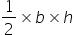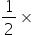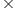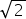Maths-
General
Easy

Question

# An isosceles right angled triangle has area 8 cm2 . Find the length of its hypotenuse.Hint:

## The correct answer is: 5.64 cm

### We know that base and height have equal length in right angled isosceles triangle.Let base = height = xIt is given that Area of the triangle = 8 cm2= 8x2 = 8x2 = 16 ⇒ x = 4 cmSo, base = 4 cm = heightUsing Pythagoras theorem,Hypotenuse2 = Base2 + Height2 = 42 + 42 = 282Hypotenuse = 4cm(= 1.41)= 5.64 cm#### With Turito Foundation.#### Get an Expert Advice From Turito.